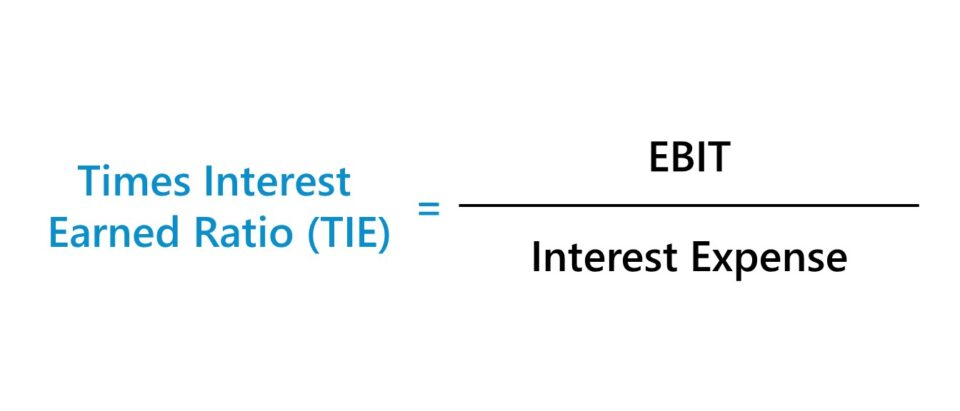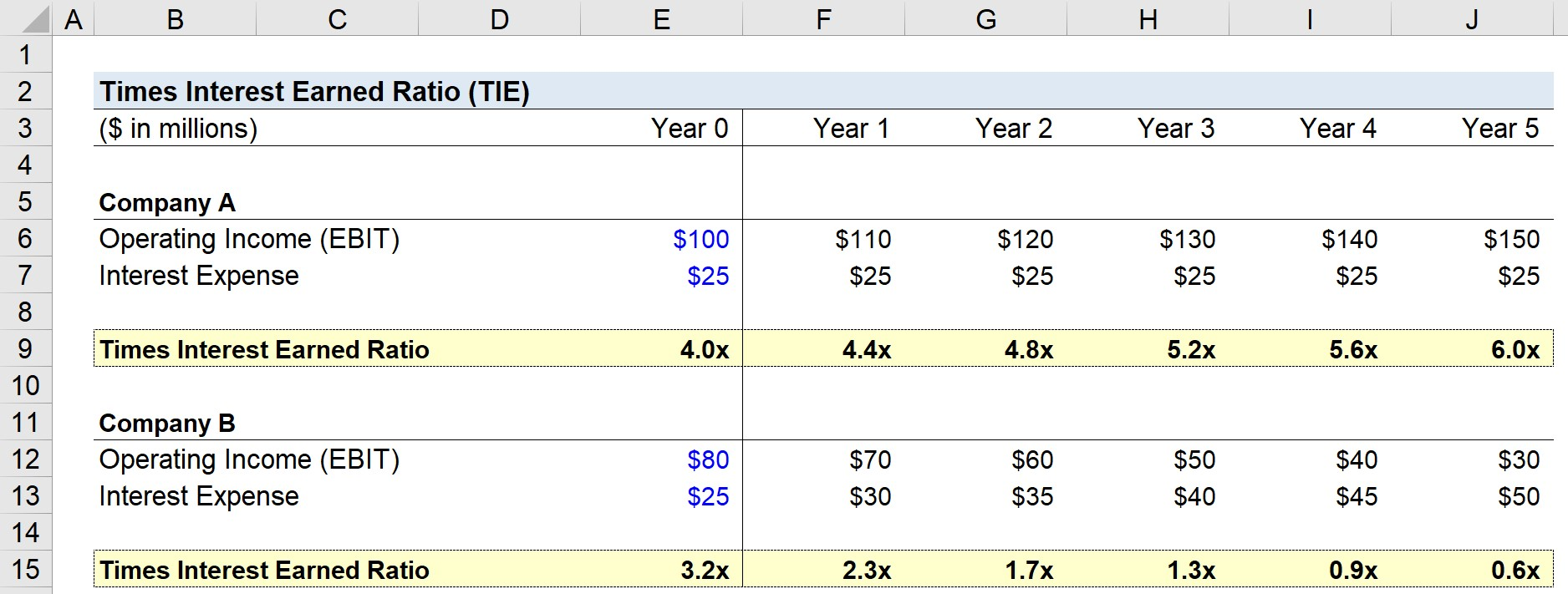Welcome to Wall Street Prep! Use code at checkout for 15% off.# Times Interest Earned Ratio (TIE)

Guide to Understanding the Times Interest Earned Ratio (TIE)## How to Calculate Times Interest Earned Ratio (TIE)?

The times interest earned ratio (TIE) compares the operating income (EBIT) of a company relative to the amount of interest expense due on its debt obligations.

Conceptually identical to the interest coverage ratio, the TIE ratio formula consists of dividing the company’s EBIT by the total interest expense on all debt securities.

## Times Interest Earned Ratio Formula (TIE)

The formula for calculating the times interest earned (TIE) ratio is as follows.

Times Interest Earned Ratio (TIE) = EBIT ÷ Interest Expense

The resulting ratio shows the number of times that a company could pay off its interest expense using its operating income.

Alternatively, other variations of the TIE ratio can use EBITDA as opposed to EBIT in the numerator.

## What is a Good TIE Ratio?

As a general rule of thumb, the higher the TIE ratio, the better off the company is from a risk standpoint.

• Higher TIE Ratio → The company likely has plenty of cash to service its interest payments and can continue to re-invest into its operations to generate consistent profits. If a company has a high TIE ratio, this signifies its creditworthiness as a borrower and the capacity to withstand underperformance due to the ample cushion (to satisfy its debt obligations) provided by its cash flows.
• Low TIE Ratio → On the other hand, a lower times interest earned ratio means that the company has less room for error and could be at risk of defaulting. Companies with lower TIE ratios tend to have sub-par profit margins and/or have taken on more debt than their cash flows could handle.

While there aren’t necessarily strict parameters that apply to all companies, a TIE ratio above 2.0x is considered to be the minimum acceptable range, with 3.0x+ being preferred.

But once a company’s TIE ratio dips below 2.0x, it could be a cause for concern – especially if it’s well below the historical range, as this potentially points towards more significant issues.

## Times Interest Earned Ratio Calculator (TIE)

We’ll now move to a modeling exercise, which you can access by filling out the form below.Submitting...

## 1. Income Statement Assumptions

In this exercise, we’ll be comparing the net income of a company with vs. without growing interest expense payments.

For Company A, we’ll be using the following listed assumptions:

• Operating Income (EBIT) in Year 0 = \$100m
• Interest Expense in Year 0 = \$25m
• EBIT Growth = \$10m / Year
• Interest Expense Growth = \$0m

Next, for Company B, we’ll be using the following listed assumptions:

• Operating Income (EBIT) in Year 0 = \$80m
• Interest Expense in Year 0 = \$25m
• EBIT Growth = – \$10m / Year
• Interest Expense Growth = \$5m / Year

## 2. Operating Income Calculation Analysis (EBIT)

Here, Company A is depicting an upside scenario where the operating profit is increasing while interest expense remains constant (i.e. straight-lined) throughout the projection period.

In contrast, Company B shows a downside scenario in which EBIT is falling by \$10m annually while interest expense is increasing by \$5m each year.

Given the decrease in EBIT, it’d be reasonable to assume that the TIE ratio of Company B is going to deteriorate over time as its interest obligations rise simultaneously with the drop-off in operating performance.

## 3. Times Interest Earned Ratio Calculation Example (TIE)

To calculate the times interest earned ratio, we simply take the operating income and divide it by the interest expense.

For example, Company A’s TIE ratio in Year 0 is \$100m divided by \$25m, which comes out to 4.0x.

• Times Interest Earned Ratio (TIE), Year 0 = \$100 million / \$25 million = 4.0x

See below for the completed output for both companies. We can see the TIE ratio for Company A increase from 4.0x to 6.0x by the end of Year 5. In contrast, for Company B, the TIE ratio declines from 3.2x to 0.6x in the same time horizon.

In closing, we can compare and see the different trajectories in the times interest earned ratio (TIE). For a lender deciding whether to provide financing to a potential borrower or not, as well as the terms associated with the lending package if applicable, Company A would be far more likely to receive favorable terms.Step-by-Step Online Course

### Everything You Need To Master Financial Modeling

Enroll in The Premium Package: Learn Financial Statement Modeling, DCF, M&A, LBO and Comps. The same training program used at top investment banks.Inline FeedbacksLearn Financial Modeling Online

Everything you need to master financial and valuation modeling: 3-Statement Modeling, DCF, Comps, M&A and LBO.

X

The Wall Street Prep Quicklesson Series

7 Free Financial Modeling Lessons

Get instant access to video lessons taught by experienced investment bankers. Learn financial statement modeling, DCF, M&A, LBO, Comps and Excel shortcuts.